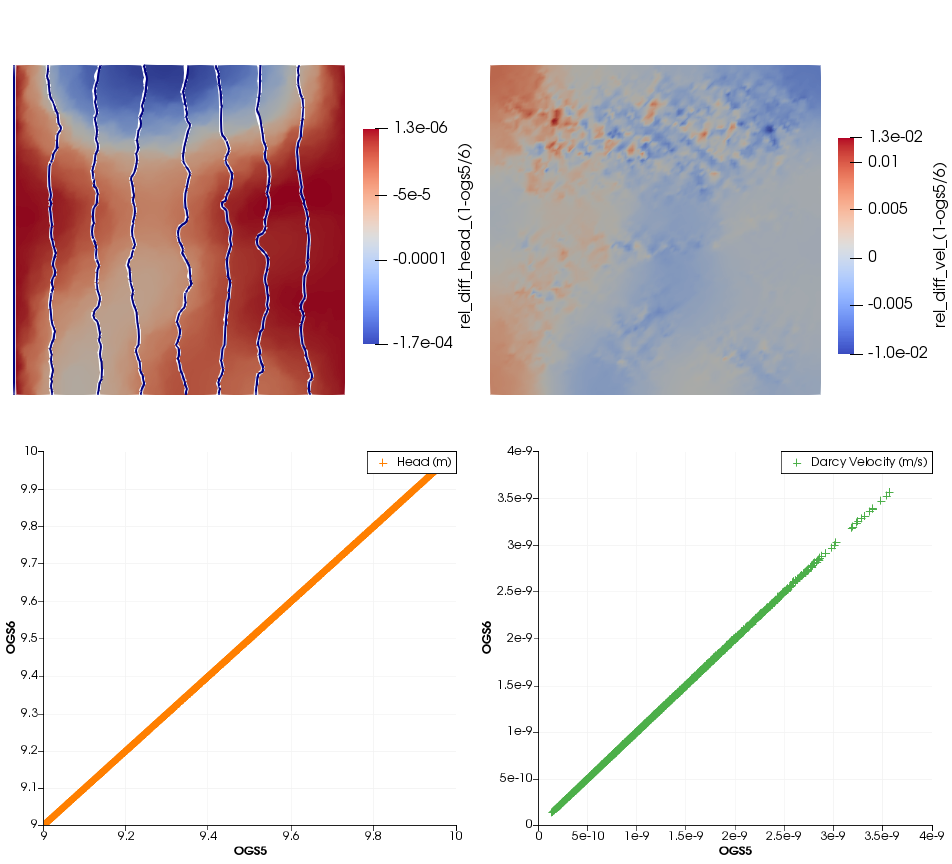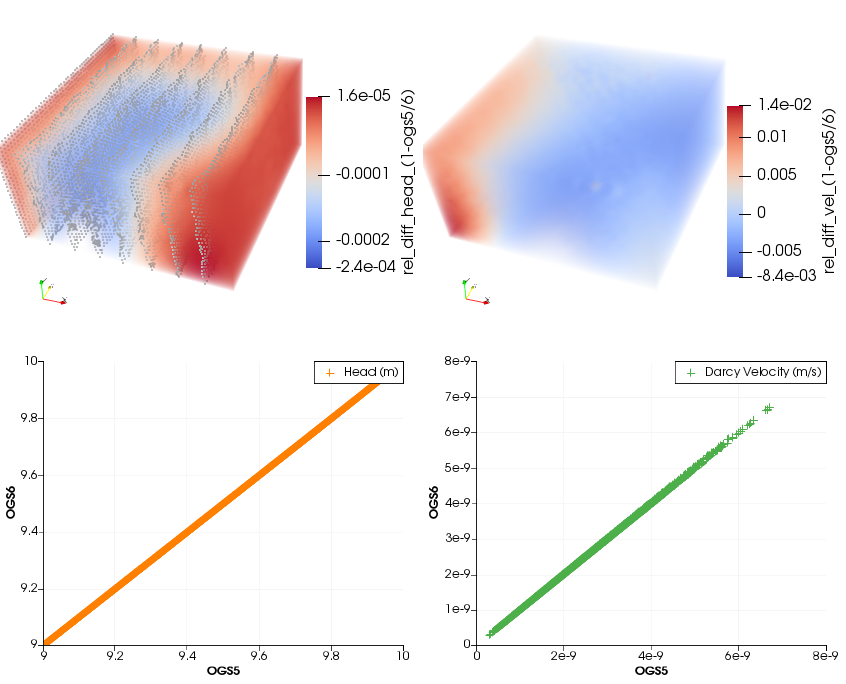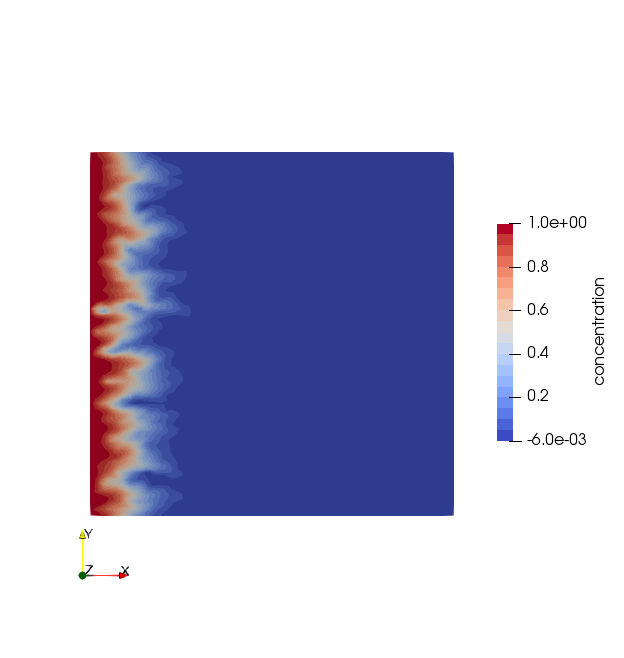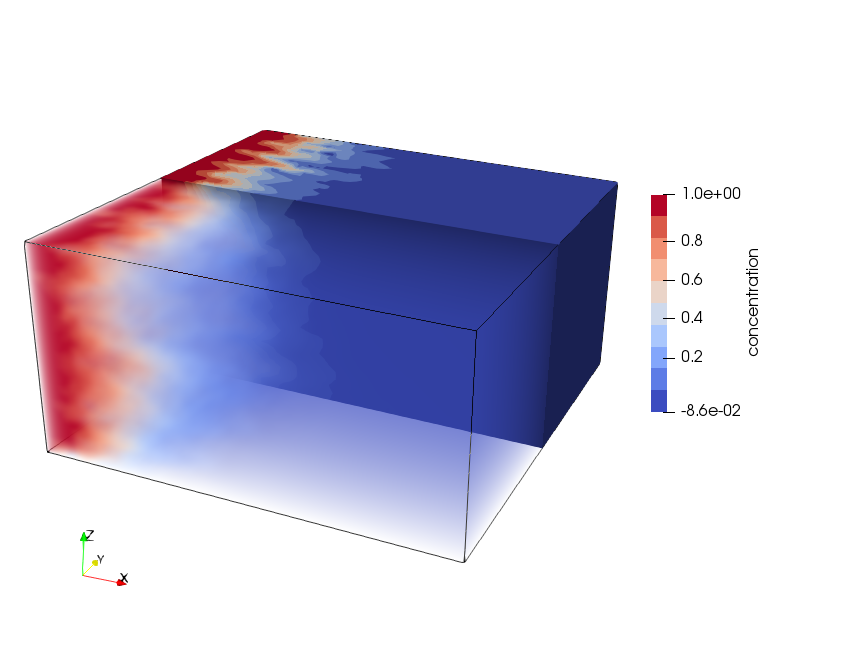# Heterogeneous Saturated Mass Transport

## Overview

This example involves the usage of a cell-based heterogeneous parameterization for the parameter “intrinsic permeability”. We show a 2D and a 3D setup and compared the results to those from OGS-5; the original examples are described in chapter “5.2 Groundwater flow in a heterogeneous medium” in Kolditz et al. (2012).

We extended the setup to show mass transport in the heterogeneous medium for testing.

## Problem description

The setups are steady-state for flow, with an extent of a $100$ m x $100$ m horizontal plane for the 2D setup and a $100$ m x $100$ m x $50$ m cube for the 3D setup. Mesh elements have side lengths of $1$ m. The initial conditions are hydrostatic and concentration $c=0$. The boundary conditions are translated into equivalent hydrostatic pressure values from hydraulic heads $h_{left}=10$ m and $h_{right}=9$ m and for concentration $c_{left}=1$, $c_{right}=0$ for left and right sides, respectively. All other sides are defined as no-flow (Zero-Neumann).

Porosity is $0.01$, specific storage is $0$, fluid density is $1000$ kg$\cdot$m$^{-3}$, dynamic viscosity is $10^{-3}$ Pa$\cdot$s, molecular diffusion coefficient is $2\cdot 10^{-9}$ m$^2\cdot$s$^{-1}$, dispersivities are longitudinal $1$ m and transverse $0.1$ m. The heterogeneous parameter fields of intrinsic permeability are shown in the figures below; creation of the tensor field is documented here.

### Model results

The comparison of velocity and hydraulic head are shown below. The numerical results of OGS-6 fit very well to the OGS-5 results with relative differences for velocity in the order of ca $10^{-2}$ and for hydraulic head in $10^{-4}$.Relative differences of 2D simulation results between OGS-5 and OGS-6. On the top left figure, white lines represent hydraulic head values of OGS-5, blue lines of OGS-6.Relative differences of 3D simulation results between OGS-5 and OGS-6. On the top left figure, grey dots represent hydraulic head values of OGS-6.

The mass transport simulation results (figures below) show an expected heterogeneous mass front moving through the domain.Concentration distribution at simulation time $1e8$ s for the 2D setup.Concentration distribution at simulation time $1e8$ s for the 3D setup.

## Literature

Kolditz, O., Görke, U.-J., Shao, H., Wang, W., 2012. Thermo-Hydro-Mechanical-Chemical Processes in Porous Media: Benchmarks and Examples, Lecture notes in computational science and engineering. Springer. ISBN: 3642271766.

This article was written by Marc Walther. If you are missing something or you find an error please let us know.
Generated with Hugo 0.117.0 in CI job 387530 | Last revision: November 7, 2023